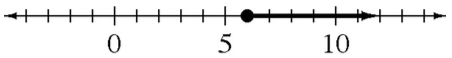### Home > INT2 > Chapter 9 > Lesson 9.1.1 > Problem9-8

9-8.

Solve the inequalities below. Show your solutions on a number line.

1. $6x-1<11$

Solve as an equation to find the boundary point. Then check points in each region to determine which region is the solution.

1. $\frac{1}{3}x\ge2$1. $9\left(x-2\right)>18$

$x>4$

1. $5-\frac{x}{4}\le\frac{1}{2}$

Start by multiplying both sides of the inequality by $4$.